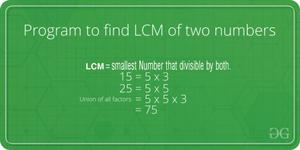Open In App
Related Articles

# Program to find LCM of two numbersLCM (Least Common Multiple) of two numbers is the smallest number which can be divided by both numbers.

For example, LCM of 15 and 20 is 60, and LCM of 5 and 7 is 35.

A simple solution is to find all prime factors of both numbers, then find union of all factors present in both numbers. Finally, return the product of elements in union.

An efficient solution is based on the below formula for LCM of two numbers ‘a’ and ‘b’.

```   a x b = LCM(a, b) * GCD (a, b)

LCM(a, b) = (a x b) / GCD(a, b)```

We have discussed function to find GCD of two numbers. Using GCD, we can find LCM.

Below is the implementation of the above idea:

## C++

 `// C++ program to find LCM of two numbers``#include ``using` `namespace` `std;` `// Recursive function to return gcd of a and b``long` `long` `gcd(``long` `long` `int` `a, ``long` `long` `int` `b)``{``  ``if` `(b == 0)``    ``return` `a;``  ``return` `gcd(b, a % b);``}` `// Function to return LCM of two numbers``long` `long` `lcm(``int` `a, ``int` `b)``{``    ``return` `(a / gcd(a, b)) * b;``}`` ` `// Driver program to test above function``int` `main()``{``    ``int` `a = 15, b = 20;``    ``cout <<``"LCM of "` `<< a << ``" and "``         ``<< b << ``" is "` `<< lcm(a, b);``    ``return` `0;``}`

## C

 `// C program to find LCM of two numbers``#include ` `// Recursive function to return gcd of a and b``int` `gcd(``int` `a, ``int` `b)``{``    ``if` `(a == 0)``        ``return` `b;``    ``return` `gcd(b % a, a);``}` `// Function to return LCM of two numbers``int` `lcm(``int` `a, ``int` `b)``{``    ``return` `(a / gcd(a, b)) * b;``}` `// Driver program to test above function``int` `main()``{``    ``int` `a = 15, b = 20;``    ``printf``(``"LCM of %d and %d is %d "``, a, b, lcm(a, b));``    ``return` `0;``}`

## Java

 `// Java program to find LCM of two numbers.``import` `java.io.*;``public` `class` `Test``{``    ``// Recursive method to return gcd of a and b``    ``static` `int` `gcd(``int` `a, ``int` `b)``    ``{``        ``if` `(a == ``0``)``            ``return` `b;``        ``return` `gcd(b % a, a);``    ``}``    ` `    ``// method to return LCM of two numbers``    ``static` `int` `lcm(``int` `a, ``int` `b)``    ``{``        ``return` `(a / gcd(a, b)) * b;``    ``}``    ` `    ``// Driver method``    ``public` `static` `void` `main(String[] args)``    ``{``        ``int` `a = ``15``, b = ``20``;``        ``System.out.println(``"LCM of "` `+ a +``                           ``" and "` `+ b +``                      ``" is "` `+ lcm(a, b));``    ``}``}`

## Python3

 `# Python program to find LCM of two numbers` `# Recursive function to return gcd of a and b``def` `gcd(a,b):``    ``if` `a ``=``=` `0``:``        ``return` `b``    ``return` `gcd(b ``%` `a, a)` `# Function to return LCM of two numbers``def` `lcm(a,b):``    ``return` `(a ``/``/` `gcd(a,b))``*` `b` `# Driver program to test above function``a ``=` `15``b ``=` `20``print``(``'LCM of'``, a, ``'and'``, b, ``'is'``, lcm(a, b))` `# This code is contributed by Danish Raza`

## C#

 `// C# program to find LCM``// of two numbers.``using` `System;``class` `GFG {``    ` `    ``// Recursive method to``    ``// return gcd of a and b``    ``static` `int` `gcd(``int` `a, ``int` `b)``    ``{``        ``if` `(a == 0)``            ``return` `b;``        ``return` `gcd(b % a, a);``    ``}``    ` `    ``// method to return``    ``// LCM of two numbers``    ``static` `int` `lcm(``int` `a, ``int` `b)``    ``{``        ``return` `(a / gcd(a, b)) * b;``    ``}``    ` `    ``// Driver method``    ``public` `static` `void` `Main()``    ``{``        ``int` `a = 15, b = 20;``        ``Console.WriteLine(``"LCM of "` `+ a +``         ``" and "` `+ b + ``" is "` `+ lcm(a, b));``    ``}``}` `// This code is contributed by anuj_67.`

## PHP

 ``

## Javascript

 ``

Output

`LCM of 15 and 20 is 60`

Time Complexity: O(log(min(a,b))
Auxiliary Space: O(log(min(a,b))

Another Efficient Approach: Using conditional for loop

## C++

 `// C++ program to find LCM of 2 numbers``#include ``using` `namespace` `std;` `// Function to return LCM of two numbers``int` `LCM(``int` `a, ``int` `b)``{``    ``int` `greater = max(a, b);``    ``int` `smallest = min(a, b);``    ``for` `(``int` `i = greater; ; i += greater) {``        ``if` `(i % smallest  == 0)``            ``return` `i;``    ``}``}` `// Driver program to test above function``int` `main()``{``    ``int` `a = 10, b = 5;``    ``cout << ``"LCM of "` `<< a << ``" and "``         ``<< b << ``" is "` `<< LCM(a, b);``    ``return` `0;``}`

## Java

 `// Java program to find LCM of 2 numbers` `import` `java.util.Scanner;` `public` `class` `LCM {``    ``public` `static` `void` `main(String[] args)``    ``{``        ``Scanner sc = ``new` `Scanner(System.in);``        ``int` `a = ``10``;``        ``int` `b = ``5``;``        ``int` `lcm = findLCM(a, b);``        ``System.out.println(``"LCM of "` `+ a + ``" and "` `+ b``                           ``+ ``" is "` `+ lcm);``        ``sc.close();``    ``}``    ``// Function to return LCM of two numbers``    ``public` `static` `int` `findLCM(``int` `a, ``int` `b)``    ``{``        ``int` `greater = Math.max(a, b);``        ``int` `smallest = Math.min(a, b);``        ``for` `(``int` `i = greater;; i += greater) {``            ``if` `(i % smallest == ``0``)``                ``return` `i;``        ``}``    ``}``}`

## Python3

 `# Python program to find LCM of two numbers` `# Function to return LCM of two numbers``def` `LCM(a, b):``    ``greater ``=` `max``(a, b)``    ``smallest ``=` `min``(a, b)``    ``for` `i ``in` `range``(greater, a``*``b``+``1``, greater):``        ``if` `i ``%` `smallest ``=``=` `0``:``            ``return` `i` `# Driver program to test above function``if` `__name__ ``=``=` `'__main__'``:``    ``a ``=` `10``    ``b ``=` `5``    ``print``(``"LCM of"``, a, ``"and"``, b, ``"is"``, LCM(a, b))`

## C#

 `// C# program to find LCM of 2 numbers``using` `System;` `class` `LCMProgram``{` `  ``// Function to return LCM of two numbers``  ``static` `int` `LCM(``int` `a, ``int` `b)``  ``{``    ``int` `greater = Math.Max(a, b);``    ``int` `smallest = Math.Min(a, b);``    ``for` `(``int` `i = greater;; i += greater) {``      ``if` `(i % smallest == 0)``        ``return` `i;``    ``}``  ``}` `  ``// Driver program to test above function``  ``static` `void` `Main()``  ``{``    ``int` `a = 10, b = 5;``    ``Console.WriteLine(``"LCM of "` `+ a + ``" and "` `+ b``                      ``+ ``" is "` `+ LCM(a, b));``  ``}``}`

## Javascript

 `// Javascript program to find LCM of two numbers` `// Function to return LCM of two numbers``function` `LCM(a, b){``    ``let greater = Math.max(a, b);``    ``let smallest = Math.min(a, b);``    ``for``(let i = greater; i <= a*b; i+=greater){``        ``if``(i % smallest == 0){``            ``return` `i;``        ``}``    ``}``}` `// Driver program to test above function``let a = 10;``let b = 5;``console.log(``"LCM of"``, a, ``"and"``, b, ``"is"``, LCM(a, b));`

Output

`LCM of 10 and 5 is 10`

Time Complexity: O(min(a,b))
Auxiliary Space: O(1)